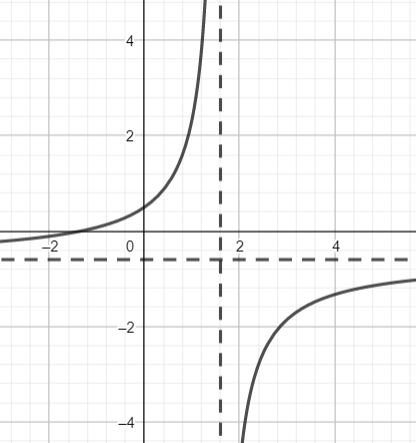# Given the rational function. f(x)= \frac {3x+4}{-5x+8}. Determine the following: a. Where the...

## Question:

Given the rational function. {eq}f(x)= \frac {3x+4}{-5x+8} {/eq}. Determine the following:

a. Where the vertical asymptote occurs?

b. At what point {eq}x {/eq} intercept occurs?

c. At what point {eq}y {/eq} intercept occurs?

d. Where the non vertical asymptote occurs?

e. Draw the graph for the given function.

## Properties of rational functions

This example illustrates how to determine various properties of the graph of a rational polynomial function.

The example shows how to determine the intercepts with the coordinate axes, plus the equations of any asymptotes.

Part a

The vertical asymptote occurs when the denominator is zero.

Hence:

{eq}-5x + 8 = 0 \\ \Rightarrow x = \dfrac{8}{5}. {/eq}

Part b

The x intercept occurs when y = 0.

So:

{eq}\dfrac {3x+4}{-5x+8} = 0 \\ \Rightarrow 3x + 4 = 0 \\ \Rightarrow x = -\dfrac{4}{3}. {/eq}

So the x intercept is at {eq}(-\dfrac{4}{3}, 0). {/eq}

Part c

The y intercept occurs when x = 0.

This gives:

{eq}y= \dfrac {3(0)+4}{-5(0)+8} = \dfrac{1}{2}. {/eq}

So the y intercept is at {eq}(0, \dfrac{1}{2}). {/eq}

Part d

The non-vertical asymptote can be found by considering the highest powers of x in the numerator and denominator.

So, as {eq}\displaystyle x_{\to \infty}, {/eq} {eq}\dfrac {3x+4}{-5x+8} \to \dfrac{3x}{-5x} = -\dfrac{3}{5}. {/eq}

Hence the non-vertical asymptote is {eq}y = -\dfrac{3}{5}. {/eq}

Part d

Below is a sketch of the graph of the function: